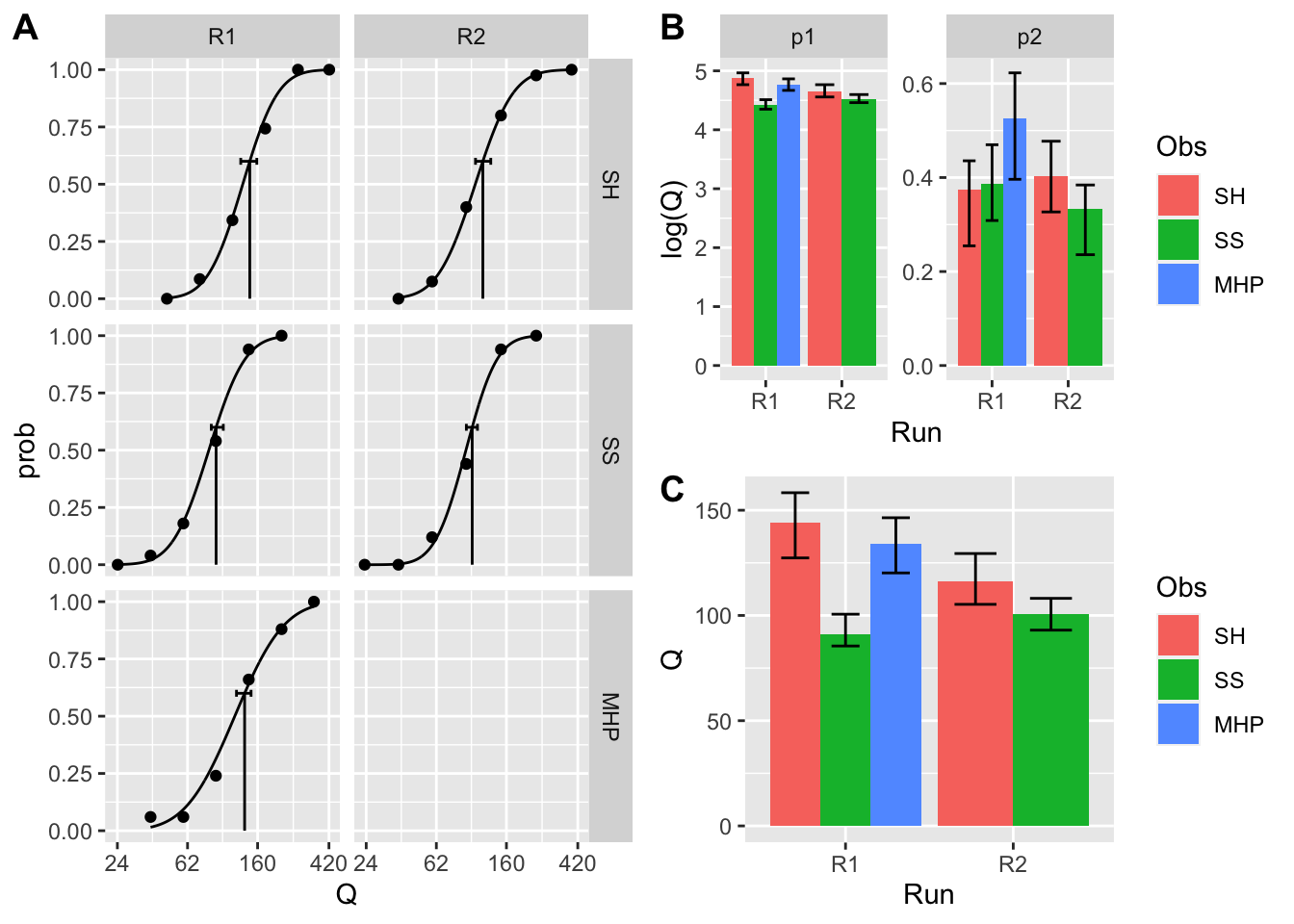To fit psychometric functions using the logarithm of the independent variable, log = TRUE should be added to quickpsy. To illustrate it, we use the data from the classic experiment of Hecht, Shlaer and Pirenne (1942) that is included in the MPDiR package associated to the book of Knoblauch and Maloney (2012).

library(cowplot)
library(quickpsy)
library(MPDiR) #contains HSP

HSP <- HSP %>% mutate(k = round(N * p / 100)) # to calculate the number of 'yes'
fit <- quickpsy(HSP, Q, k, N, prob = .6, grouping = .(Run, Obs), log = T)

pa <- fit %>% plot(xpanel = Run, ypanel = Obs)
pb <- fit %>% plotpar()
pc <- fit %>% plotthresholds()
pbc <- plot_grid(pb, pc, ncol =1, labels = c('B', 'C'))
plot_grid(pa, pbc, labels = 'A')